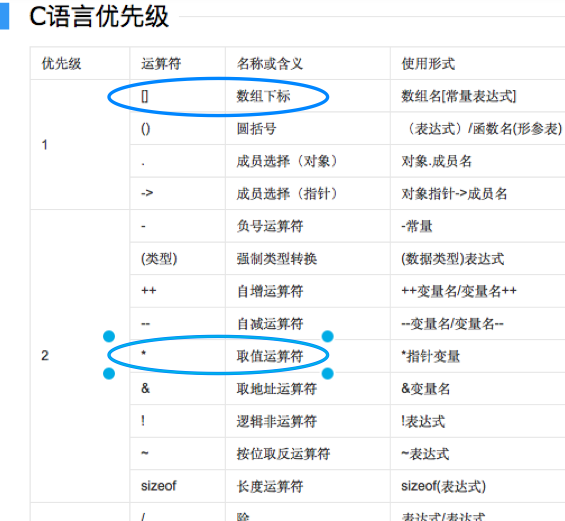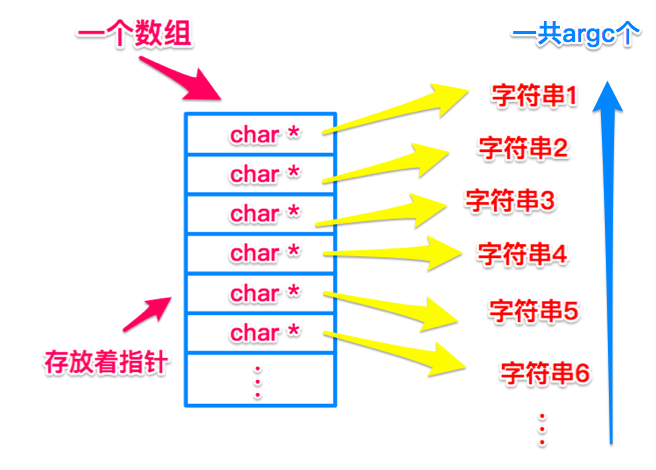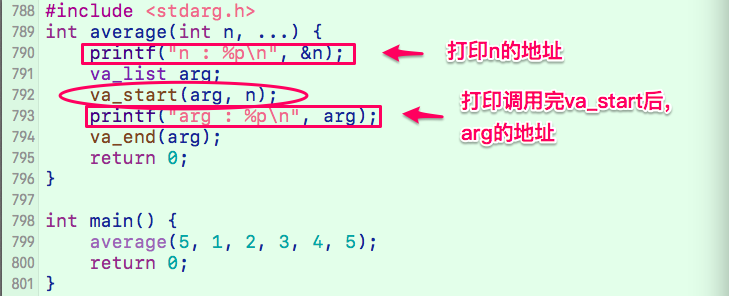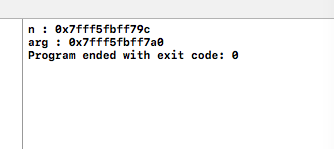# C语言| 可变参数

#include <stdio.h>
int main(int argc, const char * argv[]) {
// insert code here...
printf("Hello, World!\n");
return 0;
}


int main(int argc, const char * argv[]) {
return 0;
}


int main(int argc, char * argv[], char * envy[]) {
pragma—statements
}


argc ：一个整型变量，参数个数；
argv ：一个字符指针的数组，参数列表；
envp ：传递给该程序的环境变量列表（告诉编译器，头文件、库等信息在哪里）。char str1[] = "hi";
char* str2 = "hello";


• 第一种类型：数组是开辟了一块空间后，每一块小空间，都放入一个数据（可能是int型，可能是char型，可能是指针、结构体等等）。
• 第二种类型：一个指针，指向了一片空间，这块空间里存放着一串字符，如图所示：• 头文件
#include <stdarg.h>

• 函数名：
// 注意，参数列表里的 "..." 实际写的时候就是这么写的
// n代表之后还会传入n个参数，n后面可以没有参数，但n一定要传
int average(int n, ...) {
// ...
}

• 函数主要内容
va_list arg;
va_start(arg, n);
// ... va_arg(arg, int); // int也可为其他类型
va_end(arg);


typedef char* va_list;


void va_start ( va_list ap, prev_param );


va_start(arg, n)的功能 毫无疑问是将arg指针指向某一地址处，但我们还不清楚是指向哪里，目前我们所知道的地址只有 n 的地址，测试下和 n 的地址有何关联。// 获取类型占用的空间长度，最小占用长度为int的整数倍
#define _INTSIZEOF(n) ((sizeof(n)+sizeof(int)-1)&~(sizeof(int) - 1) )
#define va_start(ap,v) ( ap = (va_list)&v + _INTSIZEOF(v) )


v即为这里的n，ap即为这里的arg，ap = (va_list)&v，可知arg 赋为n的地址加上n的类型的大小（我写的代码中为4）。

type va_arg ( va_list ap, type );


 #define _crt_va_arg(ap,t) ( *(t *)((ap += _INTSIZEOF(t)) - _INTSIZEOF(t)) )


 void va_end ( va_list ap );

• 完整的求平均值的函数：
#include <stdio.h>
#include <stdarg.h>

int average(int n, ...) {
va_list arg; // 定义指针
int i = 0, sum = 0;
va_start(arg, n); // 指针指向第一个可变参数
for ( ; i < n; ++i) {
// 用于接收每个参数的值
sum += va_arg(arg, int);
}
va_end(arg); // 置空
return sum/n;
}

int main() {
printf("%d\n", average(5, 1, 2, 3, 4, 5));
return 0;
}


### 后续

argc >= 1，因为第一个参数就是文件名，这个参数是必备的。从第二个参数开始，是用户输入的参数。

over